ICSESolutions.com provides ICSE Solutions for Class 10 Chemistry Chapter 4 Analytical Chemistry for ICSE Board Examinations. We provide step by step Solutions for ICSE Chemistry Class 10 Solutions Pdf. You can download the Class 10 Chemistry ICSE Textbook Solutions with Free PDF download option.

## ICSE Class 10 Chemistry Chapter 4 Analytical Chemistry Solutions

### Short Questions

Question 1: What are the use of sodium and ammonium hydroxide in analytical chemistry ?
Answer: Sodium hydroxide and ammonium hydroxide are used in analytical chemistry to precipitate insoluble metal hydroxide.

Question 2: Out of the following metallic ions : Al3+, Ca2+, Cu2+, Pb2+ and Zn2+; which one forms a white hydroxide which dissolves in an excess of either aqueous sodium hydroxide or ammonium hydroxide ?

Question 3: Which reagent can be used to distinguish a solution containing a lead salt from a solution containing a zinc salt ?

Question 4: A metal, whose alloy is used in the construction of aircrafts, in the powdered form was added to sodium hydroxide solution, a colourless gas was evolved and after the reaction was over, the solution was colourless.
(i) Name the powdered metal added to sodium hydroxide solution.
(ii) Name the gas evolved.
Answer: (i) Aluminium (ii) Hydrogen (iii) Sodium aluminate, (NaAlO2).

Question 5: State the colour of the precipitate observed when caustic soda solution is added to the following solutions (prepared in water):
(i) Copper sulphate crystals (ii) Ferrous sulphate crystals
(iii) Ferric chloride crystals (iv) Lead nitrate crystals
(v) Zinc chloride crystals (vi) Calcium chloride
(vii) Zinc sulphate.
Answer: (i) Blue (ii) Dull green (iii) Reddish-brown
(iv) White (v) White (vi) White curdy
(vii) White gelatinous.

Question 6: The questions (i) to (v) refer to the following salt solutions listed A to F :
A. Copper nitrate B. Iron (II) sulphate.
C. Iron (III) chloride D. Lead nitrate
E. Magnesium sulphate F. Zinc chloride.
(i) Which two solutions will give a white precipitate when treated with dilute Hydrochloric acid followed by Barium chloride solution?
(ii) Which two solutions will give a white precipitate when treated with dilute Nitric acid followed by Silver nitrate solution?
(iii) Which solution will give a white precipitate when either dilute Hydrochloric acid or dilute Sulphuric acid is added to it?
(iv) Which solution becomes a deep /inky blue colour when an excess Ammonium hydroxide is added to it?
(v) Which solution gives a white precipitate with excess Ammonium hydroxide solution?
Answer: (i) B and E (Iron III sulphate and magnesium sulphate).
(ii) C and F (Iron III chloride and zinc chloride) (iii) D (lead nitrate)
(ii) A (copper nitrate) (v) F (Zinc chloride)

Question 7: What do you observe when caustic soda solution is added to the following solutions first a little and then in excess :
(i) FeCl3 (ii) Al2(SO4)3, (iii) ZnSO4 (iv) Pb(NO3)2 (v) CuSO4.
Also, give balanced chemical equations.
Answer: (i) A reddish-brown ppt. of ferric hydroxide is obtained which is insoluble in excess of caustic soda solution.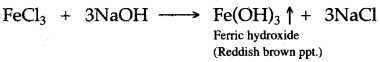(ii) A gelatinous white ppt. of aluminium hydroxide is obtained which is soluble in excess of caustic soda solution.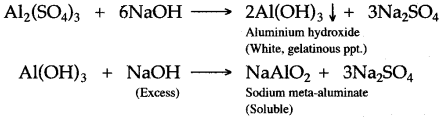(iii) A gelatinous white ppt. of zinc hydroxide is obtained which is soluble in excess of caustic soda solution.(iv) A white ppt. of lead (II) hydroxide is obtained which is soluble in excess of caustic soda solution.(v) A pie blue ppt. of copper (II) hydroxide is obtained which is insoluble in excess of caustic soda solution.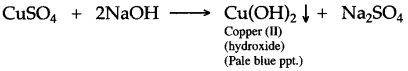Question 8: (i) What are amphoteric metals ?. Describe their reactions to hot caustic alkali.
(ii) What are amphoteric oxides? Why these oxides react with NaOH?
Answer: (i) Those metals which react with both alkalies as well as acids are called amphoteric metals e.g., Zn, Sn, Al etc. They react with caustic alkalies like NaOH, KOH on heating and liberates H2 gas. e.g.,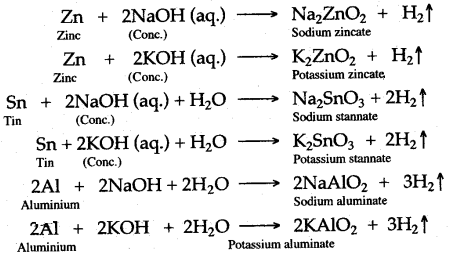(ii) Those oxides which react with both acids, as well as bases, are called amphoteric oxides. Oxides of amphoteric metals like Zn, Sn, Al, etc. react With strong alkalies like NaOH to form complex salt and water, e.g.,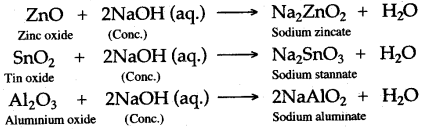### Figure/Table Based Questions

Question 1: Sodium hydroxide solution is added first in a small quantity, then in excess to the aqueous salt solution of copper (II) sulphate, zinc nitrate, lead nitrate, calcium chloride, and iron (III) sulphate. Copy the following table and write the colour of the precipitate in (i) to (v) and the nature of the precipitate (soluble/insoluble) in (vi) to (x).

 Aqueous salt solution Colour of ppt. when NaOH is added in a small quantity Nature of ppt. (soluble/ insoluble) when NaOH is added in excess Copper (II) sulphate (i) (vi) Zinc nitrate (ii) (vii) Lead nitrate (iii) (viii) Calcium chloride (iv) (ix) Iron (III) sulphate (v) (x)

 Aqueous salt solution Colour of ppt. when NaOH is added in a small quantity Nature of ppt. (soluble/ insoluble) when NaOH is added in excess Copper (II) sulphate Blue Insoluble Zinc nitrate White Soluble Lead nitrate White Soluble Calcium chloride White Insoluble Iron (III) sulphate Reddish Brown Insoluble

Question 2: Three test tubes contain calcium nitrate solution, zinc nitrate solution, and lead nitrate solution respectively. Each solution is divided into two portions. Describe the effect of:
(i) Adding sodium hydroxide solution to each portion in turn till it is in excess.
(ii) Adding ammonium hydroxide to each portion till it is in excess.

 Solution Effect of adding sodium hydroxide solution Effect of adding ammonium hydroxide solution Small amount In excess Small amount In excess Calcium nitrate A white precipitate. No change. No precipitate. No change. Zinc nitrate A white ppt. White ppt. dissolves, gives a colourless solution. A white ppt. A white ppt. dissolves to give a colour­less solution. Lead nitrate A white ppt. White ppt. dissolves to give a colourless solution. A white ppt. No change.

Question 3: The following table shows the tests a student performed on four aqueous solutions A, B, C and D. Write down on your answer sheet the observations (i) to (iv) that were made.

 Test Observations Conclusions (i) To solution A, calcium nitrate solution and sodium hydroxide solution were added. (i) A contains Ca2+ ions (ii) To solution B sodium hydroxide solution was added (ii) B contains Fe3+ ions (iii) To solution C ammonium hydroxide was added slowly till in excess (iii) C contains Cu2+ ions (iv) To solution D Lead nitrate solution and sodium hydroxide solution were  added (iv) D contains Pb2+ ions

(i) White ppt. of Calcium hydroxide is formed.
(ii) Reddish-brown ppt. of Fe(OH)3 is formed.
(iii) Pale blue ppt. is formed which gives the deep blue solution with excess ammonium hydroxide.
(iv) A white ppt. of Lead hydroxide AgCl is formed.

Question 4: Sodium hydroxide solution is added to the solutions containing the ions mentioned in list X. Y gives the details of the precipitate. Match the ions with their coloured precipitates.

 List X List Y (i) Pb2+ A. Reddish brown (ii) Fe2+ B. White insoluble in excess (iii) Zn2+ C. Dirty green (iv) Fe3+ D. White soluble in excess (v) Cu2+ E. White soluble in excess (iv) Ca2+ F. Blue

Answer: (i) Pb2+ ⟶ White soluble in excess (ii) Fe2+ ⟶ Dirty green
(iii) Zn2+ ⟶ White soluble in excess (iv) Fe2+ ⟶ Reddish brown
(v) Cu2+ ⟶ Blue (vi) Ca2+ ⟶ White insoluble in excess.

### Chemical Tests

Question: How will you distinguish between the following pairs of compounds:
1.Zinc nitrate solution and Calcium nitrate solution.
2. Sodium nitrate solution and sodium chloride solution.
3. Iron (III) chloride solution and Copper Chloride solution.
4. Iron (II) sulphate solution and copper (II) sulphate solution.
5. Zinc nitrate solution and calcium nitrate solution.
6. Iron (II) chloride and Iron (III) chloride solution.
1. Add NaOH solution in excess to the two solutions. The one in which white ppt. initially formed dissolves in excess of NaOH solution is Zn(NO3)2 solution and the other is Ca(NO3)2 solution.
2. Add freshly prepared ferrous sulphate solution to the two solutions. Then by the side of the test tube, pour cone, sulphuric acid to each slowly. The one in which brown ring appears is sodium nitrate solution while the other is sodium chloride solution.
3. Add NaOH solution to both solutions. The one which gives a reddish-brown ppt. is an iron(III) chloride solution and the one which gives blue ppt. is copper chloride solution.
4. When sodium hydroxide solution is added to iron (II) sulphate solution, a dirty green precipitate is formed.
When sodium hydroxide solution is added to copper (II) sulphate solution, light blue precipitate is formed.
5. When ammonium hydroxide is added to zinc nitrate solution, a white precipitate is formed. The white precipitate dissolves when excess of ammonium hydroxide is added.
When ammonium hydroxide is added to calcium nitrate solution, no visible reaction occurs even with the addition of excess of NH4OH.
6. When sodium hydroxide is added to Iron (II) chloride, a dirty green precipitate of Fe(OH)2 is formed.
FeCl2 + 2 NaOH   ⟶  Fe (OH)2 ↓ + 2NaCl
When sodium hydroxide is added to iron (III) chloride, reddish-brown precipitate is formed
FeCl3 + 2NaOH   ⟶   Fe(OH)3 + 3NaCl

### Balancing/Writing the Chemical Equations

Question: Write balanced chemical equations to show the reactions of the following :
1. Aluminium and caustic potash solution.
2. Aluminium oxide and sodium hydroxide.
3. Aluminium oxide and potassium hydroxide.
4. Zinc oxide and potassium hydroxide.
5. Zinc is heated with sodium hydroxide solution.
6. Zinc oxide dissolves in sodium hydroxide.
7. Caustic soda solution and Zinc oxide
8. Caustic soda solution and Aluminium oxide
9. Caustic soda solution and Lead monoxide
10. Action of KOH on CuSO4
11. Action of KOH on CaSO4
12. Action of KOH on ZnSO4
13. Action of KOH on Fe2(SO4)3.
14. Action of sodium hydroxide on freshly precipitated aluminium hydroxide.
15. Zinc oxide is treated with sodium hydroxide solution.